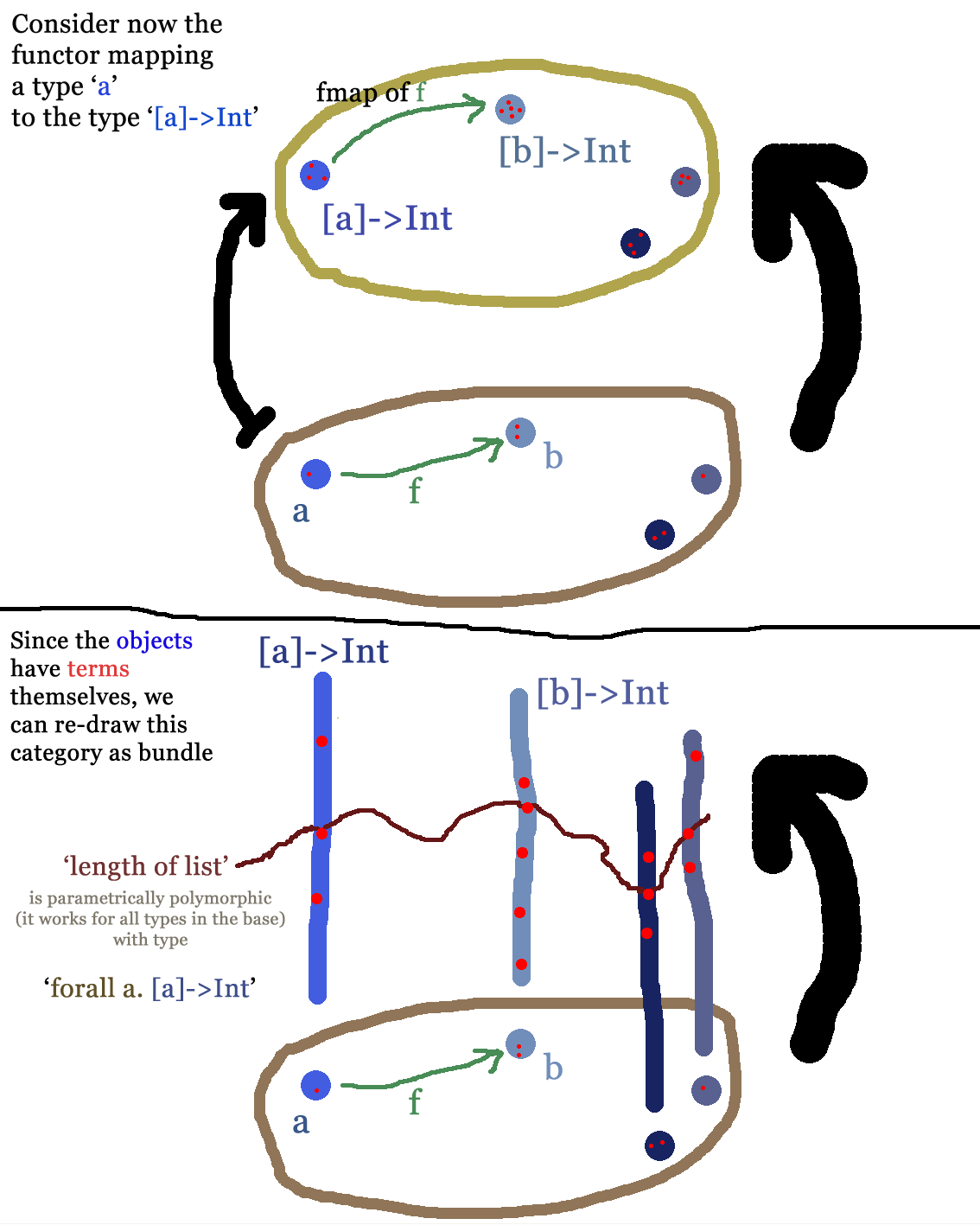# What are type system examples of local gauge transformation- and field strength-like objects?

This is essentially a follow up motivated by this answer to my question about the gauge transformation interpretation of identity types.

A field $$\psi:\mathcal M\to\mathbb C^n$$ is a section of the $\mathbb C^n$-bundle over the spacetime manifold $\mathcal M$. We have a local gauge transformations $$\psi(x)\mapsto \psi'(x):=U(x)\,\psi(x)\ :\ (\mathcal M\to \mathbb C^n)\to(\mathcal M\to \mathbb C^n).$$

Now consider a language with type polymorphism and the class $M$ of all it's types whose elements can be put into a list. Let $\Psi$ be the polymorphic function which, for every type $X\in M$, maps an $X$-list to an integer, namely its length. For example, using Haskell syntax, if $X=\mathrm{Bool}$, then $\Psi_\mathrm{Bool}\left([\mathrm{True},\mathrm{True},\mathrm{False}]\right) = 3$. In System F notation, we have

$$\Psi:\forall X.\left([X]\to\mathrm{Int}\right).$$

The gauge transformations should correspond to maps

$$u\ :\ \forall X.\left([X]\to\mathrm{Int}\right)\ \longrightarrow\ \forall X.\left([X]\to\mathrm{Int}\right).$$

I could come up with some $u$'s, for example mapping the length function $\Psi$ to a map $\Psi':=u\,\Psi$ which instead returns 42 times the length of a list. But that would be, in physics terms, a global gauge transformation because it's not sensitive to the type $X$. I think, given that the only invariant of a finite dimensional vector space is its cardinality, it shouldn't be possible to construct a local transformation in this case. What would be a hands-on example for a local gauge transformation in this sense?

Moreover, I wanted to draw an everyday life parallel to identity types. Well first there is the minor obstacle that the above transformation can't be given by an expression in most language, as types are usually not first class objects. I guess this design choice is made because otherwise type inference would be spoiled. In homotopy type theory you have realization of "types are terms too" (via n-categories?) and then it's possible. But in any case, I still can't quite pin down the specification when a type is an identity type. I understand "identity" for homotopy equivalent spaces and gauge invariant Lagrangians, but are there non-geometric structures, specifically programming relevant ones, which behave identical before and after the transformation?

edit: I now made two visualization of the example here and then:The question then is what a sensible gauge of the section in the second pic would be. (I also made two more pictures going beyond what's asked: natural transformations and monads as in Haskell.)

Btw., I know that HoTT does implement dependent types, not "just" parametrically polymorphic ones, but that should not be an obstacle.

There are a lot of rather different aspects being touched on in the question. I'll try to give some indications. But I notice that the relation of this question to actual physics is not very strong, instead the question seems to be more generally after getting a feeling for identity types in homotopy type theory (HoTT). I imagine there are other discussion groups more suitable for this kind of issues, for instance the Google-group with the (arguably unfortunate) title "HoTT amateurs" which you might think about posting questions to.

Now regarding the questions, briefly going through it from top to bottom:

first, a map $\mathcal{M} \to \mathbb{C}^n$ is a $\mathbb{C}^n$-valued function on $\mathcal{M}$. One may regard this as a section of a $\mathbb{C}^n$-fiber bundle over $\mathcal{M}$, yes, but that would then be the trivial such fiber bundle.

(Sections of non.trivial bundles may be encoded in HoTT by using dependent types. If you want to actually learn more about this, let me know and I'll expand).

Then regarding the auto-equivalences of the type of functions from lists to the natural numbers: indeed, I suppose this won't have many auto-equivalences. If one would consider not natural numbers but integers, then there would be some obvious ones (namely add +1 to each function, whose inverse would be the operation which adds -1).

Then regarding the question how to identify an identity type: one doesn't look at an arbitrary type and ask "Is this an identity type?" (One can do this, but I don't think that's what you are after.) It is rather that for a given type then one constructs the corresponding identity type. (Notice also that the identity type of some type $X$ is an $X \times X$-dependent type. )

Given the kind of questions above, it seems to me that actual applications to physics is not so much in the focus, as is getting a basic feeling for homotopy type theory as such. For that purpose I suppose there is nothing better than working through the HoTT book. (I suppose you know that already, but let me re- emphasize it anyway.)

• Thanks for the response. 1) Adding $\pm 1$ is again global as it doesn't see the types, but I get this now. 2) I want to see why gauges should be seen as identity types. You said "Notably when the homotopy type theory is equipped with the additional axiom of differential cohesion, then one can "differentiate" identity types." Is there something we get from HoTT, which we don't have if we, even more directly, set up a suitable topos for our physics? I.e. is there a reason why types actually are an advantage here, apart from the meta advantage that many different people are interested in it now? – Nikolaj-K Mar 23 '14 at 17:36
• To answer your question: yes, in HoTT there are gauge transformations, which are not present in an ordinary topos. For instance in cohesive HoTT there is a type BU(1)_conn of electromagnetic gauge fields. This does not exists in any plain topos (1-topos). For X a manifold, then a function X --> BU(1)_conn is an electromagnetic gauge field on X and a homotopy between two such maps is a gauge transformation between these field configurations. This gauge/homotopy theoretic aspect is not present in an ordinary topos. – Urs Schreiber Mar 25 '14 at 13:25